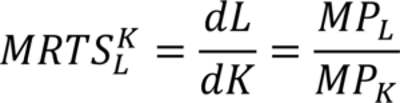Economics

# What is MILTS?

The marginal rate of technical substitution (MRTS) can be defined as, keeping constant the total output, how much input 1 have to decrease if input 2 increases by one extra unit. In other words, it shows the relationship between inputs, and the trade-offs amongst them, without changing the level of total output. When using common inputs such as capital (K) and labour (L), the MRTS can be obtained using the following formula:The MRTS is equal to the slope of isoquants. In the adjacent figure, you can see three of the most common kinds of isoquants.

The first one has an MRTS those changes along the curve and will tend to zero when diminishing the quantity of L and to infinite when diminishing the quantity of K.

In the second graph, both inputs are perfect substitutes, since the lines are parallel and the MRTS = 1, that is the slope has an angle of 450 with each axis. When considering different substitutes inputs, the slope will be different and the MRTS can be defined as a fraction, such as 1/2, 1/3, and so on. For perfect substitutes, the MRTS will remain constant.

Lastly, the third graph represents complementary inputs. In this case, the horizontal fragment of each indifference curve has an MRTS=0 and the vertical fractions an MRTS=0.